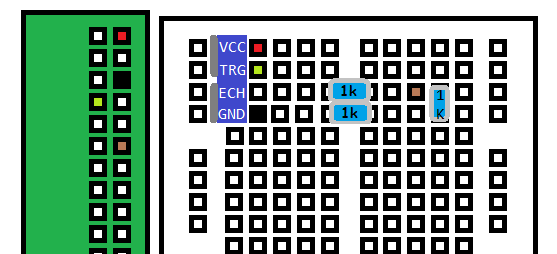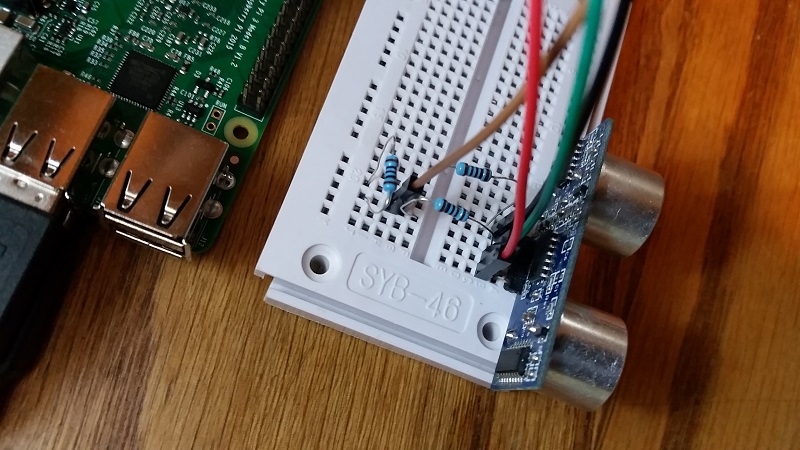## Input from a Sensor via GPIO - Raspberry Pi tutorial

Hello and welcome to part 7 of the Raspberry Pi tutorial series. In this tutorial, we're going to introduce a new sensor, the HC-SR04 ultrasonic distance sensor, along with handling GPIO input.

The HC-SR04 distance sensor measures distance based on emitting a sound burst, and timing how long it takes to receive the echo back. Using the known constant that is the speed of sound, we can mathmematically determine the distance of any object in front of this sensor by simply measuring how much time passed while the sound waves were emitted, hit the object in front of the sensor, bounced back, and came back to the sensor.

Things you will need for this tutorial besides your Raspberry Pi:

• 4 Male-to-Female jumper wire cables
• 1 x 1K Ohm and 1 x 2K Ohm resistor, OR 3x 1K Ohm resitors...or any way to build 1k resistance then 2k resistance
• 1 x HC-SR04 ultrasonic distance sensor

The distance sensor comes with 4 pins: power, trigger, echo, and ground. The power will be hooked up to the Raspberry Pi's 5V out pin, trigger will be assigned to a GPIO pin as output, echo will be assigned to a GPIO pin as input, and ground will go to a ground pin on the Pi.

The following diagram shows the setup:Like before, the boxes represent pins on the Pi, and plugs on the breadboard. The blue portion is the actual HC-SR04 sensor plugged in, and the colored-in boxes represent the M-F jumper wires by color plugged into these spots. The blue blobs with numbers on them are the resitors. If you have a 2K resistor to go before ground, feel free to use that, I just didn't have any so I used 2 1K in series.Now you can boot up the Raspberry Pi, create a new Python file, and let's setup the code:

```import RPi.GPIO as GPIO
import time

GPIO.setmode(GPIO.BCM)
```

Same imports and initial mode as in the previous tutorial.

```TRIG = 4
ECHO = 18

GPIO.setup(TRIG,GPIO.OUT)
GPIO.setup(ECHO,GPIO.IN)
```

Here, we're going to define `TRIG` and `ECHO` as the Broadcom pin #'s that we intend to use for that part of the sensor. We do this because we need to reference both pins multiple times. If we wind up wanting to change the pin placement in the future, we just need to modify a single variable rather than a bunch and risk making a mistake!

```GPIO.output(TRIG, True)
time.sleep(0.00001)
GPIO.output(TRIG, False)

while GPIO.input(ECHO) == False:
start = time.time()
```

Above, we go ahead and issue a signal out.

```while GPIO.input(ECHO) == True:
end = time.time()

sig_time = end-start
```

Now, when we finally do get an input time, we can subtract the end time from the start time and calcuate distance:

```#CM:
distance = sig_time / 0.000058

#inches:
#distance = sig_time / 0.000148

print('Distance: {} centimeters'.format(distance))

GPIO.cleanup()
```

I chose to use metric, but I provided the imperial measurement just in case you wanted that instead. We print the distance, and then cleanup the pins.

Your output should be something like:

`Distance: 8.89959006474 centimeters`

Do note that, after about 20 degrees of angle, your distance might be very skewed since the initial bounce might miss and it might take the burst several bounces off things to come back to the sensor.

Full code for this tutorial:

```import RPi.GPIO as GPIO
import time

GPIO.setmode(GPIO.BCM)

TRIG = 4
ECHO = 18

GPIO.setup(TRIG,GPIO.OUT)
GPIO.setup(ECHO,GPIO.IN)

GPIO.output(TRIG, True)
time.sleep(0.00001)
GPIO.output(TRIG, False)

while GPIO.input(ECHO) == False:
start = time.time()

while GPIO.input(ECHO) == True:
end = time.time()

sig_time = end-start

#CM:
distance = sig_time / 0.000058

#inches:
#distance = sig_time / 0.000148

print('Distance: {} centimeters'.format(distance))

GPIO.cleanup()
```

The next tutorial:• Introduction - Raspberry Pi tutorial

• Remote Access with Raspberry Pi tutorial

• Terminal Navigation - Raspberry Pi tutorial

• Raspberry Pi Camera Module - Raspberry Pi tutorial

• GPIO (General Purpose Input Output) Pins - Raspberry Pi tutorial

• Input from a Sensor via GPIO - Raspberry Pi tutorial
• Garage Stoplight GPIO Project - Raspberry Pi tutorial